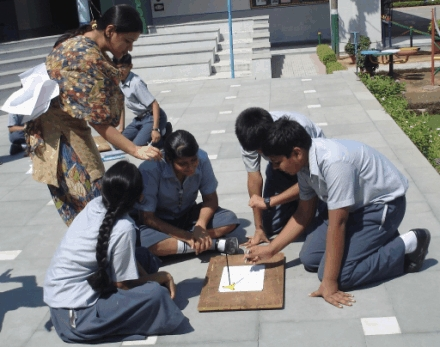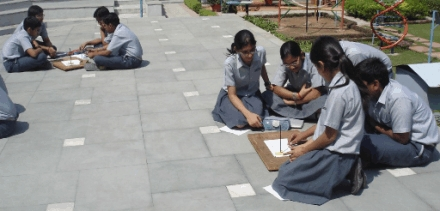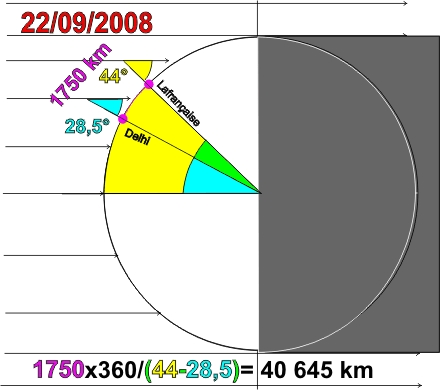Delhi

Tuesday 7 October 2008 by perbosc

New Delhi INDIA
Rukmini Devi Public School
28.38 N
77.12 E22 September2008
Metal Rod ,
gnomon : 19.7 cm
angle : 28.5°

We are grade 8 students from Rukmini Devi Public School, located in the capital city-Delhi of India (Latitude: 28.38° N and Longitude: 77.12° E) and doing this project as part of our subject “Collaborative Projects” in our school.

Firstly, our teacher explained us the set up and steps to measure the angle of the sun and circumference of the earth. We did our experiment on 22 September’ 2008 as it was Equinox on this day. We were fortunate to have sunny sky. Equinox is the time when the sun crosses the plane of the earth’s equator, making night and day of approximately equal length all over the earth.

We were divided into groups of four to measure the angle of the sun. Out of 4 groups, we submitted the data, which was considered as best in comparison to the actual data. Here’s the procedure and measurements taken by that best group:

We started our experiment at 11.45 a.m. We took metal rod of 19.7 cm as our gnomon and marked the shadow made by it after every 5 minutes of interval until the shadow started increasing. Then, we found the length of shortest shadow which came out to be 10.7 cm, which was cast by the rod at 12.15 p.m. Then by making right angle triangle between length of rod and shortest shadow of the rod, we measured the angle of the sun. The angle of the sun was measured to be 28.5°.

We took data of Grovetown Middle School situated in Georgia, U.S.A to calculate the circumference of the earth, which was done as follows:

Latitude of Delhi, India = 28.38° N
Angle of Sun measured = 28.5°

Latitude of Georgia, USA = 33.45° N
Angle of Sun measured = 33.5°

Central angle = 33.5° – 28.5° = 5.0°

Number of slices needed to make a full circle by central angle = 360 / 5 = 72.

The distance between these two places along a north-south line = 33.45°– 28.38° = 5.07°.

Now, as each angle of latitude = 111 km. Therefore, total distance between these two places along a North-South line = 5.07 x 111= 562.77 km, which can be taken as 560 km (approximately).

So if the length of the "slice" is about 560 km and there are about 72 slices, then the projected circumference is 560 x 72 = 40,320 km.

Therefore, the circumference of the earth came out to be 40,320 km, which is about 0.8% error, since the average circumference of earth is 40,008 km.

We are very excited and thrilled to know that our results are very accurate. It was a real fun and enriching experience to measure the circumference of the earth using a method that was first used by Eratosthenes.

Through this project, we came to know that angle of sun we measured was equal to the latitude of our place. As a further enhancement to the project, when we will take up this project next time, we would also find longitude by shadow measurements.

We certainly look forward to work on it in future.

Thanks,

The Noon Day Collaborative Project Group
Rukmini Devi Public School
Delhi, India